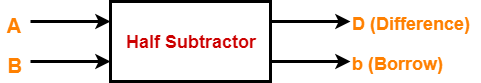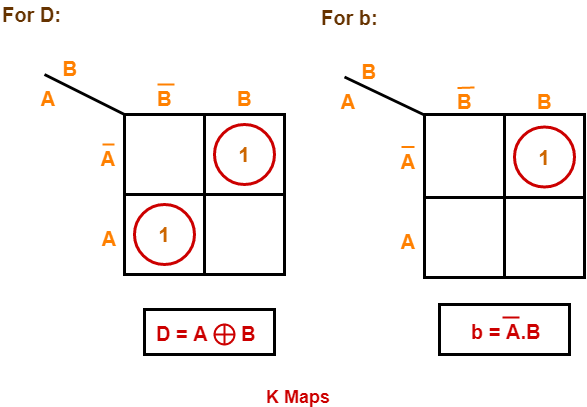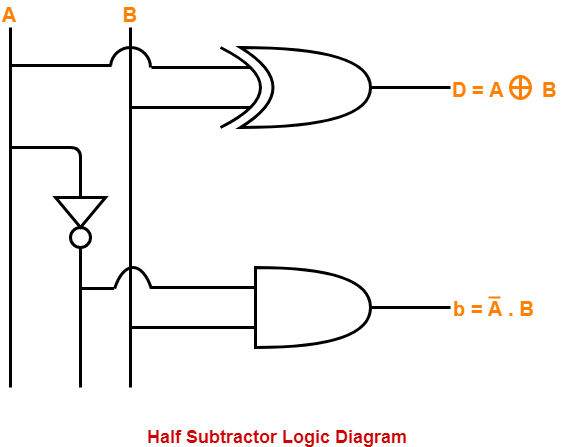# Half Subtractor | Definition | Circuit Diagram | Truth Table

## Half Subtractor-

• Half Subtractor is a combinational logic circuit.
• It is used for the purpose of subtracting two single bit numbers.
• It contains 2 inputs and 2 outputs (difference and borrow).## Half Subtractor Designing-

Half subtractor is designed in the following steps-

### Step-01:

Identify the input and output variables-

• Input variables = A, B (either 0 or 1)
• Output variables = D, b where D = Difference and b = borrow

### Step-02:

Draw the truth table-

 Inputs Outputs A B D (Difference) b (Borrow) 0 0 0 0 0 1 1 1 1 0 1 0 1 1 0 0

### Step-03:

Draw K-maps using the above truth table and determine the simplified Boolean expressions-### Step-04:

Draw the logic diagram.

The implementation of half subtractor using 1 XOR gate, 1 NOT gate and 1 AND gate is as shown below-## Limitation of Half Subtractor-

• Half subtractors do not take into account “Borrow-in” from the previous circuit.
• This is a major drawback of half subtractors.
• This is because real time scenarios involve subtracting the multiple number of bits which can not be accomplished using half subtractors.

To overcome this drawback, Full Subtractor comes into play.

To gain better understanding about Half Subtractor,

Watch this Video Lecture

Next Article- Full Subtractor

Get more notes and other study material of Digital Design.

Watch video lectures by visiting our YouTube channel LearnVidFun.

SummaryArticle Name
Half Subtractor | Definition | Circuit Diagram | Truth Table
Description
Half Subtractor is a combinational logic circuit used for the purpose of subtracting two single bit numbers. Half Subtractor Definition, Block Diagram, Truth Table, Circuit Diagram, Logic Diagram, Boolean Expression and Equation are discussed.
Author
Publisher Name
Gate Vidyalay
Publisher Logo